# Reflection (ringing) issue for transmission line at different frequencies

#### kintaro

Joined Jun 1, 2022
16
I have a circuit as below.

It consists of a data generator source with source impedance=35 Ohm. Data generated is square wave with certain rise and fall time defined in the schematic.
A transmission line has all the parameters adjusted to have the characteristic impedance of 60 Ohm at frequency=5G Hz (sine wave)
The Load impedance is 60 Ohm.

With the data rate of the source as 1G Hz (square) = 5G Hz (sine) (approximated based on same rise time and fall time), we can see that the voltage at node "V" is good because the load impedance is matched to the characteristic impedance of the line, there is no reflection to distort the signal at "V".

However if I increased the data rate of the source to 5G Hz (square) = 18G Hz (sine), the voltage at node "V" experiences ringing caused by reflection? but why is there a reflection? as the load impedance is matched to characteristic impedance of the line.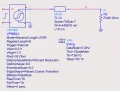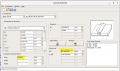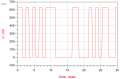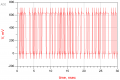To prove that the characteristic impedance remain the same between 1G Hz (square) and 5G Hz (square). I plotted the graph below.
As you can see the S11 is almost the same between 5G Hz(sine) /1G (square), and 18G Hz(sine) /5G(square).
So why is there reflection at data rate of 18G(sine)/5G Hz(square) and causes the node "V" to ring? given that the characteristic impedance of the line is the same based on the fact that S11 stays the same.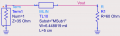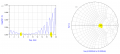Last edited: